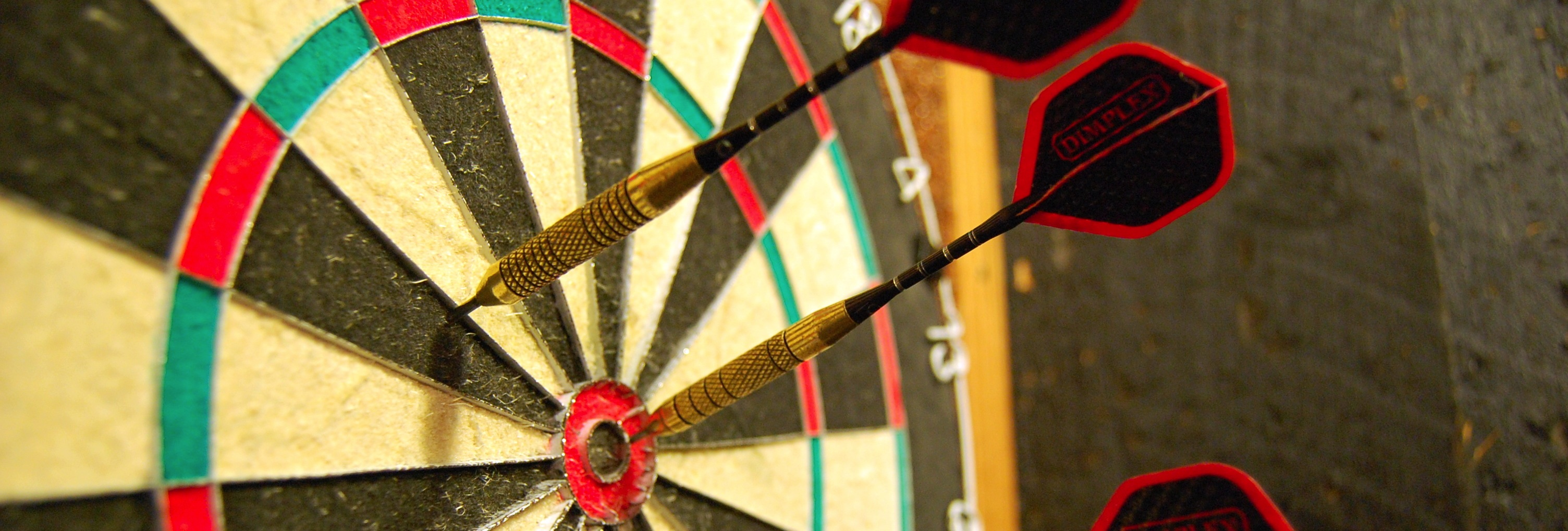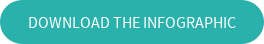When evaluating machine learning models, the validation step helps you find the best parameters for your model while also preventing it from becoming overfitted. Two of the most popular strategies to perform the validation step are the hold-out strategy and the k-fold strategy.Briefly, a validation set is a subsection of your data that you set aside for the purpose of selecting the best parameters for your model. Central to hold-out validation, the validation set is not used in the initial training of the model and provides an independent measure of the performance of the model. Once the best parameter combination of each model has been found, the model is then retrained on the full data.

Pros of the hold-out strategy: Fully independent data; only needs to be run once so has lower computational costs.

Cons of the hold-out strategy: Performance evaluation is subject to higher variance given the smaller size of the data.

K-fold validation evaluates the data across the entire training set, but it does so by dividing the training set into K folds - or subsections - (where K is a positive integer) and then training the model K times, each time leaving a different fold out of the training data and using it instead as a validation set. At the end, the performance metric (e.g. accuracy, ROC, etc. -- choose the best one for your needs) is averaged across all K tests. Lastly, as before, once the best parameter combination has been found, the model is retrained on the full data.

Pros of the K-fold strategy: Prone to less variation because it uses the entire training set.

Cons of the K-fold strategy: Higher computational costs; the model needs to be trained K times at the validation step (plus one more at the test step).

Today, we present to you an illustration of how these two methods work: